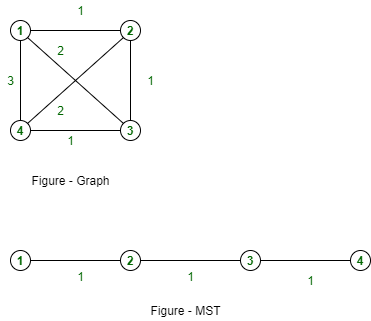# GATE | GATE CS 2020 | Question 59

Consider a graph G=(V, E), where V = { v1,v2,…,v100 }, E={ (vi, vj) ∣ 1≤ii, vj) is ∣i–j∣. The weight of minimum spanning tree of G is ________ .

Note – This question was Numerical Type.
(A) 99
(B) 100
(C) 98
(D) 101

Explanation: There are 100 vertices numbered from 1 to 100.
Edges are presents in this graphs E={ (vi, vj) ∣ 1≤i1, v2), (v2, v3), ….. (v99, v100) will have minimum weight, i.e., 1 for each edge.

As we know that, there are 99 edges are possible in minimum spanning tree of 100-vertices graph.Therefore, these edges (v1, v2), (v2, v3), ….. (v99, v100) will be spanning tree for given graph. These are 99 edges with 1 cost of each.

The weight of minimum spanning tree of G is 99*1 = 99.

Option (A) is correct.

Quiz of this Question

My Personal Notes arrow_drop_up
Article Tags :

1

Please write to us at contribute@geeksforgeeks.org to report any issue with the above content.